• 分析时间复杂度和空间复杂度 1：实现二分查找算法的递归及非递归。（分析时间复杂度及空间复杂度） 2：实现斐波那契数列的递归及非递归。（分析时间复杂度及空间复杂度） 分析尾递归 实现斐波那契数列的尾递归 ...
分析时间复杂度和空间复杂度
1：实现二分查找算法的递归及非递归。（分析时间复杂度及空间复杂度）
2：实现斐波那契数列的递归及非递归。（分析时间复杂度及空间复杂度）
分析尾递归
实现斐波那契数列的尾递归
——————–我是分割线—————–

分析时间复杂度和空间复杂度

时间复杂度：
时间复杂度实际就是一个函数，该函数计算的是执行基本操作的次
数。
算法分析的分类：算法存在最好、平均和最坏情况。
最坏情况：任意输入规模的最大运行次数(上界)
平均情况：任意输入规模的期望运行次数
最好情况：任意输入规模的最小运行次数，通常最好情况不会出现(下界)
例如：在一个长度为N的线性表中搜索一个数据x
最好情况：1次比较
最坏情况：N次比较
平均情况：N/2次比较
在实际中通常关注的是算法的最坏运行情况，即：任意输入规模N，算法
的最长运行时间。理由如下：一个算法的最坏情况的运行时间是在任意输入下的运行时间上界，对于某些算法，最坏的情况出现的较为频繁，大体上看，平均情况与最坏情况一样差。因此：一般情况下使用O渐进表示法来计算算法的时间复杂度。
时间复杂度之大O渐进表示法：
一个算法语句总的执行次数是关于问题规模N的某个函数，记为f(N)，N
称为问题的规模。语句总的执行次数记为T(N)，当N不断变化时，T(N)也
在变化，算法执行次数的增长速率和f(N)的增长速率相同。则有T(N) =
O(f(N))，称O(f(n))为时间复杂度的O渐进表示法。
一般算法O(n)计算方法：
（1）用常数1取代运行时间中的所有加法常数
（2）在修改后的运行次数函数中，只保留最高阶项
（3）如果最高阶项系数存在且不是1，则去除与这个项相乘的常数

空间复杂度：
空间复杂度是对一个算法在运行过程中临时占用存储空间大小的量度，记做S(n)=O(f(n))。比如菲波那切数列的非递归算法的时间复杂度是O(n),空间复杂度是O(1) 。而一般的递归算法就要有O(n)的空间复杂度了，因为每次递归都要存储返回信息。一个算法的优劣主要从算法的执行时间和所需要占用的存储空间两个方面衡量。

1：实现二分查找算法的递归及非递归。（分析时间复杂度及空间复杂度）

//二分查找非递归
int binarry_Search(int arr[], int len, int key)
{
int left = 0;
int right = len - 1;
while (left <= right)
{
int mid = (left&right) + ((right^left) >> 1);   //求平均值
if (key<arr[mid])
{
right = mid - 1;
}
else if (key>arr[mid])
{
left = mid + 1;
}
else
{
return mid;
}
}
return -1;
}
//时间复杂度 O(lgN)
//空间复杂度 O(1)

//递归二分查找
int recur_binarry_Search(int arr[], int left, int right, int key)
{
if (left <= right)
{
int mid = (left&right) + ((right^left) >> 1);   //求平均值
if(arr[mid] == key)
return mid;
else if (key<arr[mid])
return  recur_binarry_Search(arr, left, mid - 1, key);
else if (key>arr[mid])
return  recur_binarry_Search(arr, mid + 1, right, key);
}
else
return -1;
}
//递归二分查找时间复杂度 O(lgN)
//空间复杂度 O(lgN)

int main(){
int arr[] = { 0, 1, 2, 3, 4, 5, 6, 7, 8, 9 };
int len = sizeof(arr) / sizeof(int)-1;
printf("%d\n", recur_binarry_Search(arr, 0, len, 0));
printf("%d\n", recur_binarry_Search(arr, 0, len, 1));
printf("%d\n", recur_binarry_Search(arr, 0, len, 2));
printf("%d\n", recur_binarry_Search(arr, 0, len, 3));
printf("%d\n", recur_binarry_Search(arr, 0, len, 4));
printf("%d\n", recur_binarry_Search(arr, 0, len, 5));
printf("%d\n", recur_binarry_Search(arr, 0, len, 6));
printf("%d\n", recur_binarry_Search(arr, 0, len, 7));
printf("%d\n", recur_binarry_Search(arr, 0, len, 8));
printf("%d\n", recur_binarry_Search(arr, 0, len, 9));
printf("%d\n", recur_binarry_Search(arr, 0, len, 10));
system("pause");
return 0;
}

2：实现斐波那契数列的递归及非递归。（分析时间复杂度及空间复杂度）

//  非递归
int Fib1(int n)
{
int a = 0;
int b = 1;
if (n<2)
{
return n;
}
while (n-->1)
{
int c = a + b;
a = b;
b = c;
}
return b;
}
//时间复杂度为 O(N)
//空间复杂度为 O(1)

//递归
int Fib2(int n)
{
if (n <= 2)
return 1;
return Fib2(n - 1) + Fib2(n - 2);
}
//时间复杂度 O(2^n)
//空间复杂度 O(N)

int main(){
int num = 0;
printf("请输入num:> ");
scanf("%d", &num);
printf("%d\n", Fib1(num));
printf("%d\n", Fib2(num));
system("pause");
return 0;
}

尾递归

定义：
如果一个函数中所有递归形式的调用都出现在函数的末尾，我们称这个递归函数是尾递归的。当递归调用是整个函数体中最后执行的语句且它的返回值不属于表达式的一部分时，这个递归调用就是尾递归。尾递归函数的特点是在回归过程中不用做任何操作，这个特性很重要，因为大多数现代的编译器会利用这种特点自动生成优化的代码。
原理：
当编译器检测到一个函数调用是尾递归的时候，它就覆盖当前的活动记录而不是在栈中去创建一个新的。编译器可以做到这点，因为递归调用是当前活跃期内最后一条待执行的语句，于是当这个调用返回时栈帧中并没有其他事情可做，因此也就没有保存栈帧的必要了。通过覆盖当前的栈帧而不是在其之上重新添加一个，这样所使用的栈空间就大大缩减了，这使得实际的运行效率会变得更高。
实例：
1:实现斐波那契数列的尾递归

//尾递归
// 1 1 2 3 5 8 13 21 34 ...
int Fib3(int n, int a, int b){
if (n <2)
{
return a;
}
else
{
return Fib3(n - 1, b, a + b);
}
}
//时间复杂度为 O(N)
//空间复杂度为 S(1) 
展开全文C语言 数据结构
• 时间复杂度为O(logn) 时间复杂度为O(1) 递归实现 非递归二分查找时间复杂度为O(logn) 时间复杂度为O(1) 2、斐波那契数列 递归 时间复杂度为O(2^n) 时间复杂度为O(1) 非递归 时间复杂度为O(n) ...
1、二分查找

非递归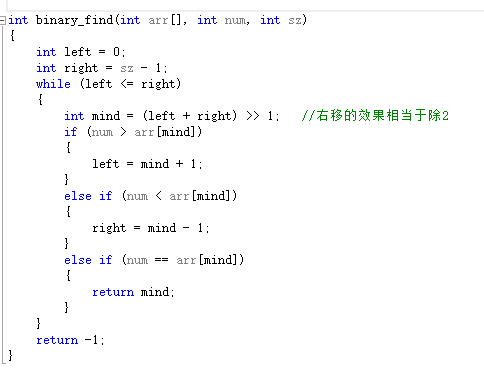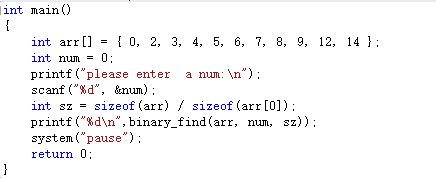非递归二分查找：
时间复杂度为O(logn)
空间复杂度为O(1)

递归实现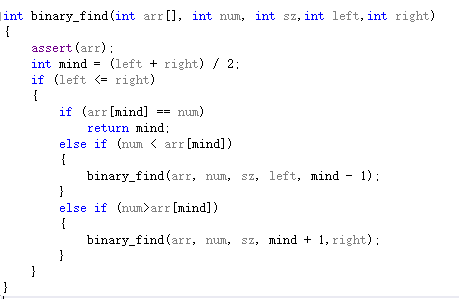非递归二分查找：
时间复杂度为O(logn)
空间复杂度为O(1)
2、斐波那契数列

递归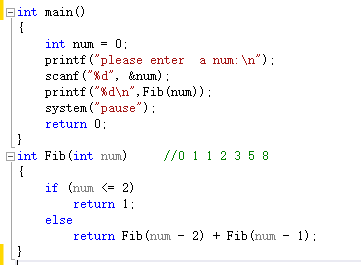时间复杂度为O(2^n)
空间复杂度为O(1)

非递归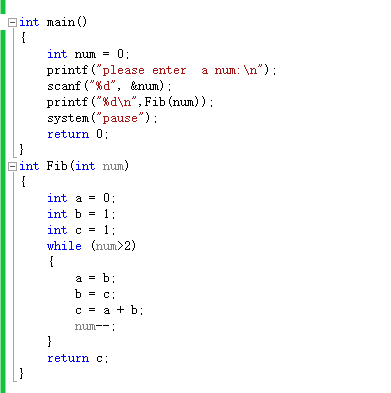时间复杂度为O(n)
空间复杂度为O(1)
总结

1、时间复杂度就是一个计算执行基本操作的次数的函数 一般算法O(n)计算方法：
<1>用时间1取代运行时间中的所有加法常数

<2>在修改后的运行次数函数中，只保留最高阶项

例如：2*n^3+n （只保留n^3,因为n^3的增长率远远大于n）

<3>如果最高阶项系数存在且不是1，则去除与这个项相乘的常数
(所以上式的2可以除去，时间复杂度为0(n^3) )

2、递归算法时间复杂度=递归总次数*每次递归次数

3、空间复杂度：函数中创建对象的个数关于问题规模函数表达式
展开全文• 一、时间复杂度：实际是指程序运行次数，而不是程序运行时间1.我们一般讨论的是最坏时间复杂度，这样做的原因是：最坏... ③递归时间复杂度=递归总次数 * 每次递归中基本操作所执行的次数 例1，代码如下：void Test2
一、时间复杂度：实际是指程序运行次数，而不是程序运行时间

1.我们一般讨论的是最坏时间复杂度，这样做的原因是：最坏情况下的时间复杂度是算法在任何输入实例上运行时间的上限，以最坏代表最全。

2.时间复杂度的书写规则：

①忽略常数项，用O(1)表示
②选取最坏时间复杂度即选取增长最快的项
③递归的时间复杂度=递归总次数 * 每次递归中基本操作所执行的次数

例1，代码如下：

void Test2(int n)
{
int iCount = 0;
for (int iIdx = 0; iIdx < 10; ++iIdx)
{
iCount++;
}
for (int iIdx = 0; iIdx < 2*n; ++iIdx)
{
iCount++;
}
for (int i = 0; i < n; ++i)
{
for (int j = 0; j < n; ++j)
{
iCount++;
}
}
}

f(n)=n^2+2n+10
则O(n)=n^2       //规则①②

例2，代码如下：

int factorial(int n)//求n的阶乘，递归法
{
if(n<=1)
return 1;
else
{
return n*factorial(n-1);
}
}

因为if()判断的两种情况对应的两个代码块中的基本操作的执行次数都为1
递归次数为n，所以O(n)=n*1=n  //规则③

例3，代码如下：

void Test4(int m, int n)// f(n,m) = 2*m*n == O(m*n)
{
int iCount = 0;
for (int i = 0; i < 2*m ; ++i)
{
for (int j = 0; j < n ; ++j)
{
iCount++;
}
}
}

f(n)=2*m*n
则O(n)=(m*n) //规则①

3 .   常用的时间复杂度有以下七种，算法时间复杂度依次增加：

O(1):常数型
O(log2 n):对数型
O(n):线性型
O(nlog2n):二维型
O(n^2):平方型
O(n^3):立方型
O(2^n):指数型

二、空间复杂度：不是计算实际占用的空间，而是计算整个算法的辅助空间单元的个数，与问题的规模没有关系(简单理解就是算法执行时创建的变量（包括临时变量）个数)

1.空间复杂度书写规则：

①忽略常数，用O(1)表示
②递归算法的空间复杂度=递归深度N*每次递归所要的辅助空间
③对于单线程来说，递归有运行时堆栈，求的是递归最深的那一次压栈所耗费的空间的个数，因为递归最深的那一次所耗费的空间足以容纳它所有递归过程。递归是要返回上一层的，所以它所需要的空间不是一直累加起来的
（简单说明单线程和多线程的区别：当你吃饭的时候电话响了，你接完电话再吃饭，这是单线程；你边吃饭边接电话，这是多线程。）

例1，代码如下：

int Sum(int N)
{
int count = 0;
for(int i = 1; i <= N; ++i)
{
count += i;
}
return count;
}

空间复杂度：O(n)=1 //规则①

例2，代码如下：

void fun(int n)
{
int arr;
if(n<0)
{
return 1;
}
else
{
return fun(n-1);
}
}

每次调用fun函数就会创建一个10个元素的整型数组，调用次数为n
空间复杂度：O(n*10)=O(n) //规则①②

三、实例解析：
1.二分查找
1>递归法：

int binary_search_recursion(int *arr,int left,int right,int num)
{
assert(arr);

if(left<=right)
{
int mid = left + ((right - left) >> 1);
if (arr[mid] > num)
{
return binary_search_recursion(arr,left,mid-1,num);
}
else if (arr[mid] < num)
{
return binary_search_recursion(arr, mid+1, right, num);
}
else
{
return mid;
}
}
else
{
return -1;
}
}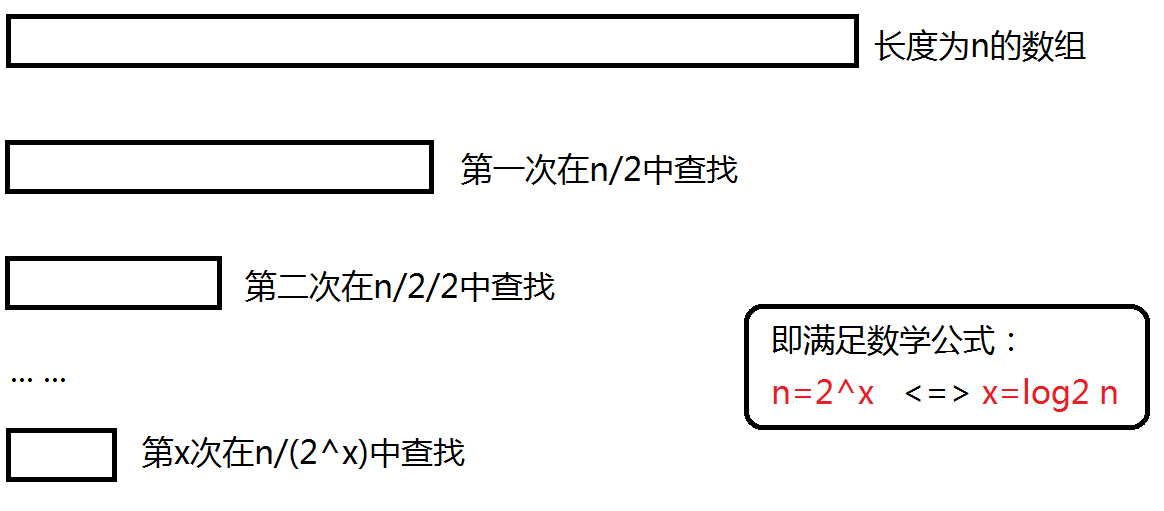递归的次数和深度都是log2 n,每次所需要的辅助空间都是常数级别的：
时间复杂度 :  O(log2 n)
空间复杂度：O(log2 n)

2>迭代法：

int binary_sreach_iteration(int *arr, int sz, int num)
{
int mid = 0;
int left = 0;
int right = sz - 1;

while (left<=right)
{
mid = (left + right)>>1;
if (arr[mid]>num)
{
right = mid - 1;
}
else if (arr[mid]<num)
{
left = mid + 1;
}
else
{
return mid;
}
}
return -1;
}

循环的基本次数是log2 n，所以:时间复杂度是O(log2 n);
由于辅助空间是常数级别的所以：空间复杂度是O(1);

2.斐波那契
1>递归法：

int fib_recursion(int n)
{
if (n <= 2)
return 1;
else
{
return fib(n - 1) + fib(n - 2);
}
}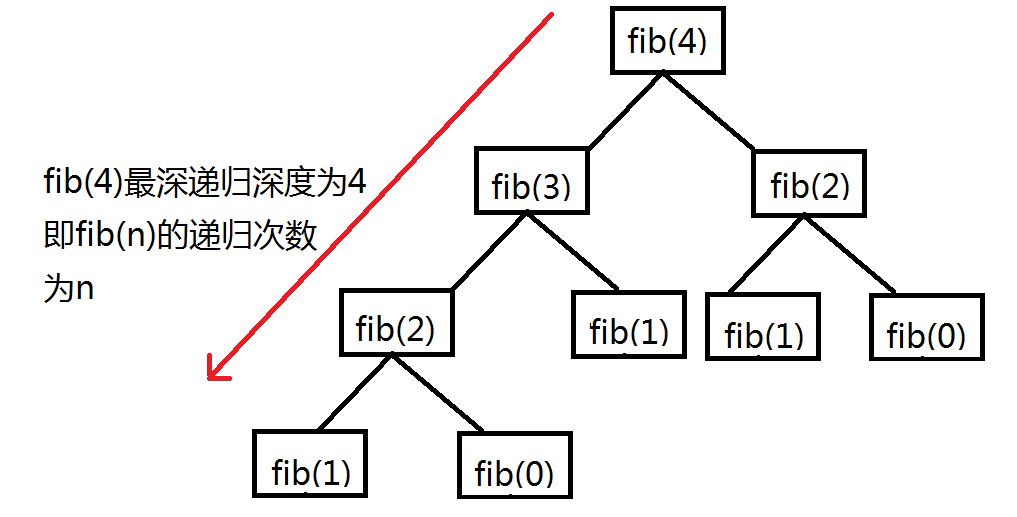循环的基本操作次数是n-1,辅助空间是n+1，所以：
时间复杂度O(2^n)
空间复杂度O(n)

2>迭代法：

int fib_iteration(int n)
{
int a = 1;
int b = 1;
int c = 1;
if (n<2)
{
return n;
}
while (n>2)
{
c = a + b;
a = b;
b = c;
n--;
}
return c;
}

循环的基本次数是n-1，所用的辅助空间是常数级别的：
时间复杂度：O(n)
空间复杂度：O(1)

展开全文空间复杂度 斐波那契
• 什么是递归前面我们对一些算法的复杂度进行了分析，但这些都是基于循环和迭代的，这一节我们会对递归的算法进行复杂度分析。首先要需要知道什么是递归递归（recursion）是函数调用自身的一个过程。举个例子，假设...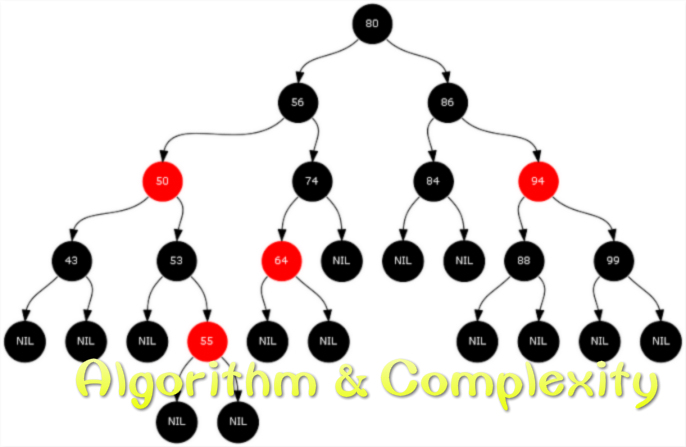什么是递归前面我们对一些算法的复杂度进行了分析，但这些都是基于循环和迭代的，这一节我们会对递归的算法进行复杂度分析。首先要需要知道什么是递归，递归（recursion）是函数调用自身的一个过程。举个例子，假设你是一个英语水平有限的人，你在读一段英文材料中遇到了某个生词，你需要查字典去了解这个单词的意思，但是只给你了英英字典，意味着你要查找的单词下方的释义也有可能是你不认识的单词，于是你又继续去查找那些你不认识的单词，而那些单词的释义有可能又出现你不认识的单词，你又继续查找，如此往复，直到你能够全部看懂为止。这样一个过程我们可以把它看做是一个递归的过程，因为查字典这个动作在不停地调用自身。试想如果你的英语水平很糟糕，你可能查了很久的字典还是没有弄明白原来那个生词是什么意思，甚至出现单词 A 用到了单词 B 做释义，单词 B 又用到了单词 A 做释义这种情况，即出现死循环。那么递归结束的条件是什么呢？显然，如果一个单词的释义你全都能看懂，那就意味着当前递归就结束了，我们把这种条件叫做初始条件（initial condition），也叫递归出口。阶乘的计算阶乘大家应该都不陌生，一个数的阶乘，所以我们自然而然想到的计算方法是从 1 到把它们乘起来，但只要我们稍微观察一下便可以得到这样的关系式。递归需要递归出口，因此这里我们规定 0 的阶乘等于 1，所以当时递归就停止了。用代码实现阶乘计算非常容易，只需要 4 行就可以搞定：def factorial(n):
if n == 0:
return 1
return n * factorial(n-1)用一个数学递推式是来表达上面的关系：我们观察可以得到这样的规律：问题的规模由原来的经过一次乘法运算后变成了，并且每经过一次乘法运算，问题的规模都会减少 1，所以要求计算阶乘的时间复杂度，我们可以推出如下的关系式：其中代表问题的规模每减少 1 就执行了一次乘法运算。我们把这样的关系式叫做递归关系式（recurrence relation），要解出这个关系式，我们需要一步一步地推导，具体来讲就是先将代入式子中得到一个新的递归关系式，再将它代入到原式子中就可以得到，一直重复这个过程，直到出现递归出口。于是我们得出计算阶乘的时间复杂度为。汉诺塔汉诺塔大家肯定都玩过，规则也很简单：有 A, B, C 三根柱子，上面穿有从大到小排列的圆盘，现在你需要借助 B 柱把 A 柱上的圆盘挪到 C 柱上，一次只能挪一个，且大的圆盘不能放在小的圆盘的上面。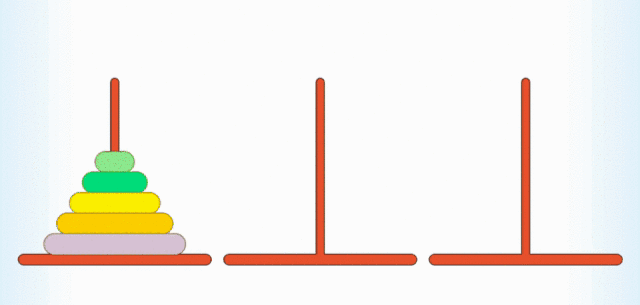汉诺塔跟递归有什么关系呢？如果你是一个细心观察的人，你就会发现，要想把个圆盘从 A 柱挪到 C 柱上，你首先要通过某种方法把个柱子从 A 挪到 B 柱上，给 A 柱上最大的圆盘“腾位置”，然后把最大的圆盘从 A 柱移动到 C 柱上，最后再通过某种方法把 B 柱上个圆盘挪到 C 柱上。至于怎么移动这个柱子，我们可以又用某种方法移动最上面的个柱子，给第二大的圆盘“腾位置”，再将个柱子移动到相应的柱子上。这样我们便可以一直递归下去，直到出现递归出口，也就是只有一个圆盘的情况。我们用几张图来直观地理解一下，假设这里有 4 个圆盘，我们可以分为三个步骤：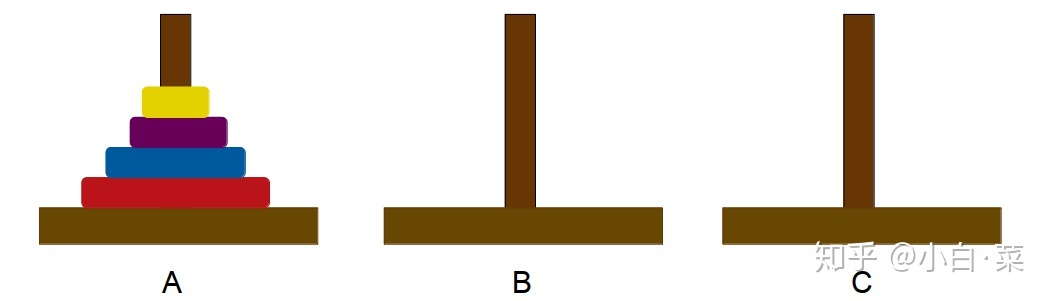步骤一：用某种方法将 3 个圆盘从 A 柱移动到 B 柱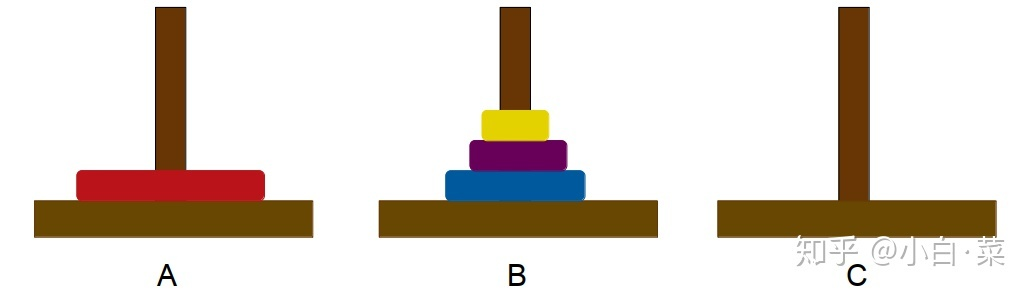步骤二：将 A 柱的圆盘移动到 C 柱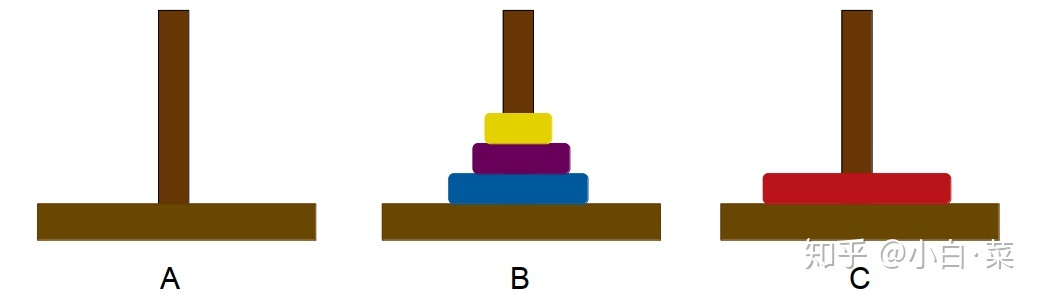步骤三：再用某种方法将 3 个圆盘从 B 柱移动到 C 柱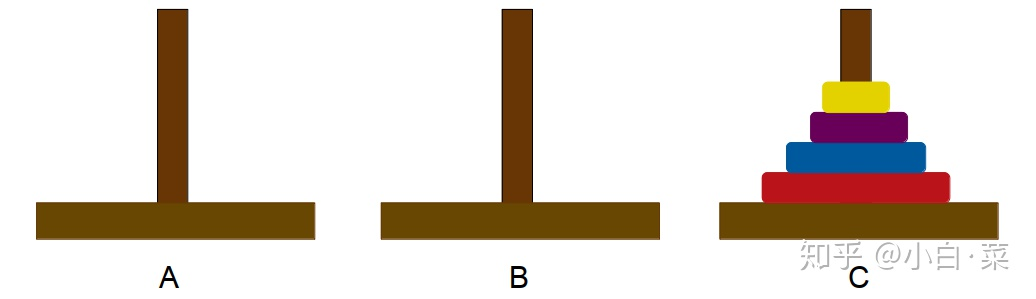那么怎样来写递归关系式呢？我们看到，要解决阶汉诺塔的问题，我们先要解决两个阶同样的问题，外加一个单次移动。所以我们的递归关系式应该这样写：我们还是采用回代的方式解出这个关系式：复杂度为，最后我们用代码来实现一下吧。def hanoi(a, b, c, n):
if n == 1:
print("{} -> {}".format(a, c))
return
hanoi(a, c, b, n-1)  # c 柱为枢纽，将 a 柱中 n - 1 个圆盘移到 b 柱上
hanoi(a, b, c, 1)  # 将待移动的圆盘数设为 1
hanoi(b, a, c, n-1)  # a 柱为枢纽，将 b 柱中 n - 1 个圆盘移到 c 柱上让我们试试n = 3时输出的结果：>>> hanoi(3)
... a -> c
... a -> b
... c -> b
... a -> c
... b -> a
... b -> c
... a -> c→本节全部代码←← 复杂度分析（非递归）| 算法与复杂度​zhuanlan.zhihu.com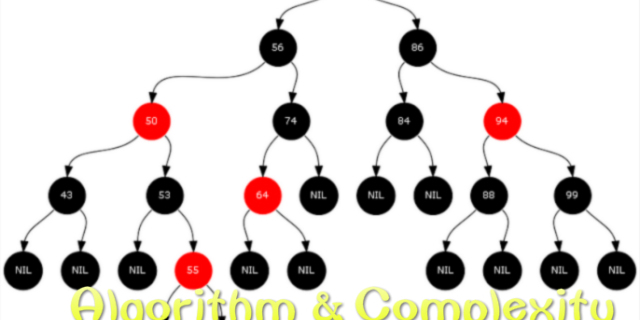→ 冒泡排序与选择排序 | 算法与复杂度​zhuanlan.zhihu.com展开全文• 递归 递归条件 自己调用自己 有结束条件 二分查找 二分查找对1~100乱序数字查找 l = list(range(1,101)) def bin_search(data_set,val): low = 0 high = len(data_set) - 1 while low <= high: mid = (low+...算法 数据结构
• 如果每次递归使问题的规模减半，而其他操作都是常数时间， 则T(N)=O(logN)。比如二分法查找，就是很棒的例子。 T(N)=T(N-1)+O(1) 若每次递归使用问题的规模减1，而其他操作是常数时间，则T(N)=O(N)。比如我前几天...
• 如何理解递归: 把递归的执行顺序，画出状态树 ...2、想尽所有可能的方法，同时比较这些时间、空间复杂度 3、找出最优的解决方案，最优的解决方案时间最少，用的内存最少，然后开始写 4、测试实验结果 ...
•  递归算法的时间复杂度递归的总次数*每次递归的数量。  递归算法的空间复杂度：递归的深度*每次递归创建变量的个数。  那什么是斐波那契额数列呢？对于菲波那切数列有典型的生兔子的的问题，在这我就不多说了...
• 1、递归 　递归的两个条件： 　（1）、调用自身 　（2）、结束条件 ...2、时间复杂度 3、空间复杂度 4、列表查找 利用递归的方法进行二分法 data = [1, 3, 6, 7, 9, 12, 14, ...
• 最烦面试官问，“为什么XX算法的时间复杂度是OO”，今后，不再惧怕这类问题。快速排序分为这么几步：第一步，先做一次partition；partition使用第一个元素t=arr[low]为哨兵，把数组分成了两个半区：左半区比t大右...
• 1.时间复杂度： 时间复杂度其实即使算法执行次数n的某个函数f(n)，进而分析f(n)随n的变化情况并确定T(n)的数量级。这里用"O"来表示数量级，时间复杂度的表达式为。 T(n)=O(f(n)); 它表示随着问题规模的...
• 二分查找： int BinarySearch(int* arr, int size, int to_find) { assert(arr); int left = 0; int right = size - 1; while (left <= right) { int mid = (left + right) / 2; if (arr[mid] < to_find...
• 任何一个分治或者递归的函数都可以算出它的时间复杂度 关键4种 二分查找:发生在一个数列本身有序的时候,要在有序的数列中找到你要的目标数,所以每次一分为2只查一边,最后时间复杂度是O(logN) 从 T(n) = T(n/2) + O(1...
• //二分查找的非递归递归实现： #include int binarry_Search(int arr[], int len, int value){ //采用左闭右闭区间方式 int left=0,right=len-1; int mid; while(left){ mid=left+((right-left)>>1); //...
• 文章最后，举例使用二分查找和斐波那契的递归和迭代方法，分别说明时间和空间复杂度。 时间复杂度： 首先要说的是，时间复杂度的计算并不是计算程序具体运行的时间，而是算法执行语句的次数。 当我们面前有多...斐波那契
•  最高项系数不为1的改为1注意：选取最坏时间复杂度即选取增长最快的项递归时间复杂度=递归总次数*每次递归中基本操作所执行是次数 空间复杂度 函数中创建对象的个数关于问题规模表达式不是计算实际占用的空间，...
• O(N^d)，d是除去递归代码外的其他运算的时间复杂度 例如，二分查找递归，将原始问题每次划分成两份，依次递归。 这个问题中，每次将问题划分成两份，所以b是2，然后依次递归左右部分，因此，子递归的调用了2次，a...
•   二分查找又称为折半查找，是一种查询效率比较高的查找方法，但是二分查找的前提是要求线性表必须采用顺序存储结构而且表中元素按照关键字有序排列。 代码如下：   该程序在循环体内的关键在于三路分支的判断...
• 时间复杂度 常数复杂度 O(1) 对数复杂度 O(log n) ... 每一递归或分治程序都可以计算出其时间复杂度 二分查找 O(log n) 二叉树查找 O(n) 在排序好的二维矩阵中进行二分查找 O(n) 归并排序 [所有排序时间复杂度一致...